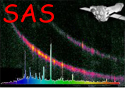XMM-Newton Science Analysis System

lcplot (lcplot-1.22.1) [xmmsas_20211130_0941-20.0.0]

## Examples

To create a postscript plot, called plot.ps, with both net and background count rates and the results of the Chi Square and Kolmogorov-Smirnov tests, from the FITS file 'lightcurve.ds', where the counts are binned up by a factor 10, a command such as,

```lcplot set="lightcurve.ds" plotdevice="/VPS" plotfile="plot.ps"
binsize=10  tests=both
```

can be used.

XMM-Newton SOC -- 2021-11-30